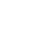# Sort an Array with a Nested for Loop using Insertion Sort in JavaScript

Insertion sort is another sorting algorithm that closely resembles how we might sort items in the physical world. We start at the second item in our collection and make the assumption that this item is a sorted list of length 1. We then compare all the items before it and determine if it needs to be "inserted" to the left or right of our item. We then move onto the second item, again comparing it to every item before it in the list, inserting those items correctly.

Because this algorithm requires two loops, one inside the other, the worst case scenario of our algorithm still requires a time complexity of `O(n^2)`. This is also an inefficient sorting algorithm, but if our list is mostly sorted already, it will perform a slight bit better than bubble sort.Fabian
~ 3 years ago

Hi kyle, I'm wandering if this algorithm could be more efficient by usign destructuring(probalbly I'm using the wrong term) than using "splice" method, I'm not sure but splice could cause another iteration in the array, isn't it?.

Therefore the code would be:

``````...
for (i = 1; i < array.length; i++) {
for (j = 0; j < i; j++) {
printArray(array)
count++

if (array[i] < array[j]) {
[array[i], array[j]] = [array[j], array[i]]
}
}
}
...``````Kyle Shevlininstructor
~ 3 years ago

Hi Fabian,

I highly doubt that splice uses loops under the hood. Arrays are very fast with operations involving indices, since they are essentially stored as hashes with each key as an index. Thus, splice is likely an O(1) operation under the hood, just making a direct copy of the items and doesn't loop through each item. For the sake of teaching the concept, this is a fine way of showing insertion sort.Fabian
~ 2 years ago

Hi Kyle, thank you for the clarification :)